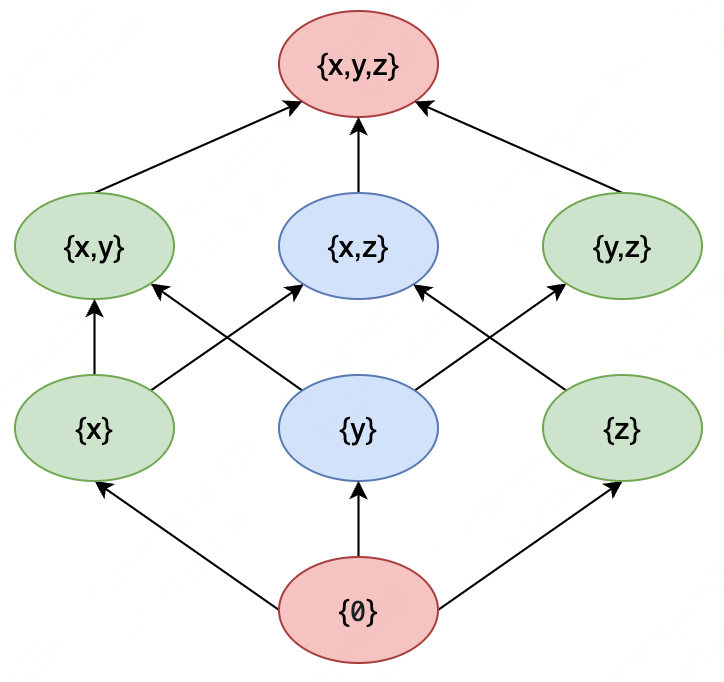# # 《程序员数学：幂集》- 该集合的所有子集

## # 二、幂集实例

``````{
{}, // (also denoted empty set ∅ or the null set)
{x},
{y},
{z},
{x, y},
{x, z},
{y, z},
{x, y, z}
}
``````
1
2
3
4
5
6
7
8
9
10## # 三、密集实现

### # 1. 方案1

``````public static List<List<Integer>> btPowerSet(int[] originalSet) {
return btPowerSetRecursive(originalSet, new ArrayList<>(), new ArrayList<>(), 0);
}

private static List<List<Integer>> btPowerSetRecursive(int[] originalSet, List<List<Integer>> allSubsets, List<Integer> currentSubSet, int startAt) {
for (int position = startAt; position < originalSet.length; position++) {
btPowerSetRecursive(originalSet, allSubsets, currentSubSet, position + 1);
currentSubSet.remove(currentSubSet.size() - 1);
}
return allSubsets;
}
``````
1
2
3
4
5
6
7
8
9
10
11
12
13

• originalSet是要求幂集的数组
• allSubsets是存储所有子集的结果集合
• currentSubSet是正在生成的当前子集
• startAt是从originalSet的哪个位置开始生成子集 它通过递归不断地在当前子集上添加元素，最终生成所有子集，并将其存储在allSubsets中。

### # 2. 方案2

``````public static List<List<Integer>> caPowerSet(int[] originalSet) {
List<List<Integer>> sets = new ArrayList<>();
for (int i : originalSet) {
int existingSetsNum = sets.size();
for (int setIdx = 0; setIdx < existingSetsNum; setIdx++) {
List<Integer> set = new ArrayList<>(sets.get(setIdx));
}
}
return sets;
}
``````
1
2
3
4
5
6
7
8
9
10
11
12
13
• 这段代码是一个用递归算法实现的求集合的幂集的Java函数。它将一个给定的整数数组作为输入，并返回所有子集的列表。
• 该函数以一个空的列表开始，每次遍历输入数组的元素并将该元素添加到现有的所有子集中，最终返回所有子集的列表。

### # 3. 方案3

``````public static List<List<Integer>> bwPowerSet(int[] originalSet) {
List<List<Integer>> subSets = new ArrayList<>();
int numberOfCombinations = (int) Math.pow(2, originalSet.length);
for (int combinationIndex = 0; combinationIndex < numberOfCombinations; combinationIndex++) {
List<Integer> subSet = new ArrayList<>();
for (int setElementIndex = 0; setElementIndex < originalSet.length; setElementIndex++) {
if ((combinationIndex & (1 << setElementIndex)) != 0) {
}
}
}
return subSets;
}
``````
1
2
3
4
5
6
7
8
9
10
11
12
13
14